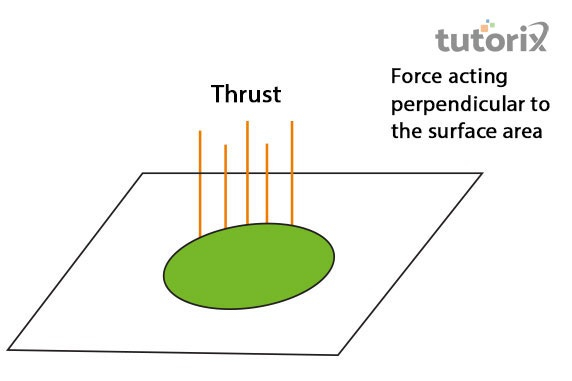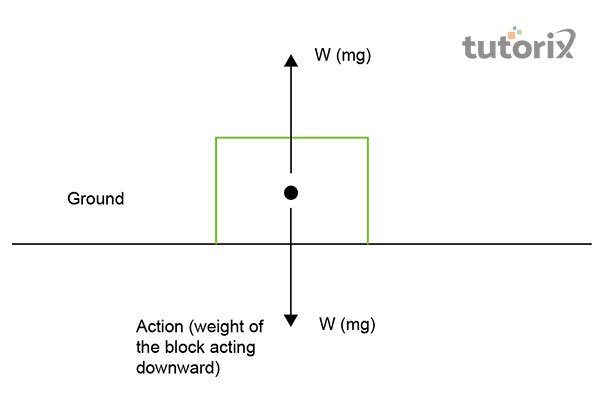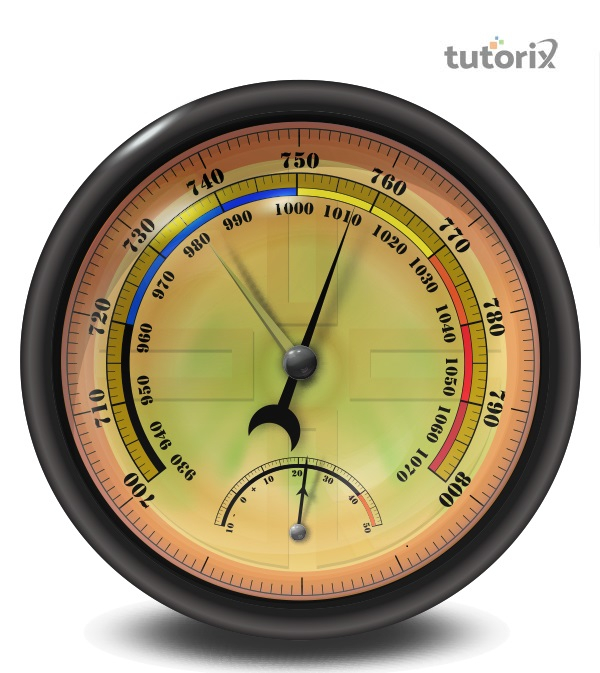# Thrust Pressure

## Introduction

Thrust is the perpendicular acting force on the surface and pressure is the exerted thrust "per unit of a body".

Newtown is the SI unit used for the calculation of the thrust of an object. According to physics, the force which is applied on a surface can regulate the object’s body movement as a perpendicular force on the surface of the following object. Additionally, thrust is the upward force which is applied on the surface of an object.

## Define Thrust

The acting strength that is vertical to the object’s surface is thrust in physics. It is conveyed by 'T'. The thrust’s SI unit is identical to the force’s SI unit. It is estimated by the "Newton" (N).

Thrust is a vector portion due to both its direction and magnitude can describe it. The applied force of an object is pressurised from the upside on the object’s source. The pressure on the "surface of the object" will make the objects undergo a "force of buoyancy" when they are submerged in a fluid (Snyder et al. 2020).

Based on the application of thrust, the objects type such as liquid or solid, and the amount of SI units can be distinctive. The up thrust is estimated in "Newton" in the international system of measuring units.Figure 1: "Definition of Thrust"

## Define Pressure

Pressure is the apple thrust per unit of the object’s surface that also plays a vital role in the situations to calculate the amount of thrust that has been applied with the SI units. An object can be moved only by applying the pressure which can be either pushed or pulled depending on the situation and the applied amount of thrust. Pressure is depending on the "surface area" where it acts. The common formula of pressure is the

$\mathrm{Pressure\:(P) = Thrust\:(T)\:/\:Area\: (A)}$

(Practically, 2022). The body pressure can be exercised from any direction and there is less specific direction.

## Example

The running trucks with heavy loads and buses maintain the wide tyres due to the more extensive scope of tyres reducing the stress on the road.Figure 2: Force and Pressure

## Factors Affecting Pressure

The exerted pressure is relying on the surface of the object where the following factors are dependable:

"The surface area in contact"

An object's pressure can increase with a decreasing amount in the "area of the surface" on which it is generally exercised and it can be vice versa.

"The direction of thrust"

An object's pressure is directly symmetrical to the object’s acting thrust. The pressure can be exerted from the acting thrust and the SI unit can apply it, which is "Newton".

Additionally, air can exert pressure in all the possible magnitude and the objects’ surface can match the direction of the moving Earth. The exerted thrust by the air beyond the surface of Earth is due to its gravitational power and it is called the "atmospheric pressure" (Knizhnik & Yim, 2021). Additionally, the "Atmospheric pressure" decreases with an enlargement in measurement from the "ground level". An instrument named "barometer" calculates thrust.

## Pressure Examples

While we are trying to cut fruit from the flat side of the knife, then less amount of pressure has been exerted on the fruit and it becomes difficult to cut. On the other hand, while using the shaper side of the knife one can easily cut the fruit as more pressure has been exerted. Another example is that camels can walk on the sand with ease as they have broad soles. As a result of that, they exert less pressure on the sand and it makes them easier to walk. In addition to that, heavier trucks are using wider tyres as it provides the wheels larger areas to distribute the pressure of the whole car.

## Pressure Exerted by AirFigure 3: Barometer

Air in the atmosphere exerts pressure in all directions. All objects on the earth feel pressure due to the atmosphere presented here. Due to gravitation, the atmosphere also exerted pressure on the objects which is called atmospheric pressure (Nationalgeographic, 2022). With an increase in altitudes, air pressure on any object gets reduced. With the help of a barometer, it can be measured. "Height of the Mercury column" is mainly used to measure the pressure of the atmosphere. At the sea level amount of pressure exerted by the "atmosphere" is about "760 mm" of mercury.

## Difference between Thrust and Pressure

The core difference that is noticed between these two terms of physics is that the force of thrust acts at a perpendicular angle of the surface area of the body, whereas the pressure refers to the force that is acted and measured based on per unit area of the object. The trust is considered a vector quantity whereas pressure is considered as a scalar quantity.

## Conclusion

The present tutorial has shed light on the concepts of physics which are pressure and thrust. In this tutorial, it has been explained when a force acts on the surface of a body in a perpendicular manner, the force is recognised as "thrust" and the measurement of such force acting per unit area of the body is considered as pressure. This explanation has been considered the main difference shared between these two concepts of physics. Furthermore, this tutorial has included the factors that affect pressure along with an exemplification of pressure.

## FAQs

Q1. What is the formula of thrust and pressure?

The formula of thrust is generally represented as the "production of pressure" and the "area which it acts on". On the contrary, the formula of pressure states that the measurement of pressure is equivalent to the area on which thrust is projected.

Q2. What is the dimension of thrust?

The “dimensional formula” of thrust is “$\mathrm{[MLT^{-2}]}$”. Based on this formula, the “dimension of impulse” will be calculated as "$\mathrm{MLT^{-1}}$"

Q3. What is the unit of pressure?

In accordance with physics, the basic unit of pressure is "the Pascal" which has been defined as the "pressure exerted by a force" of "one Newton" that stands perpendicular to an area of "one square meter".